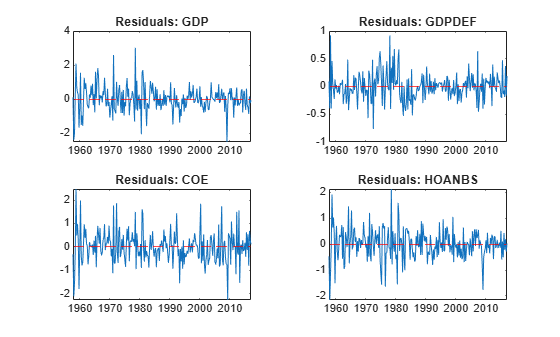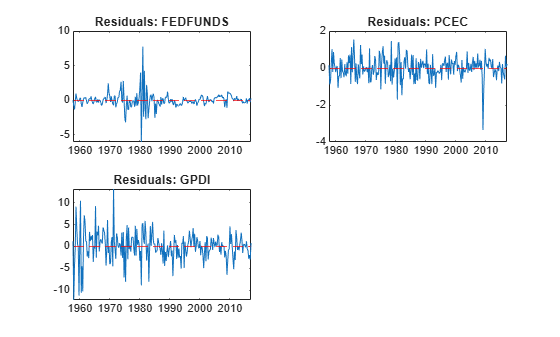# infer

Infer vector error-correction (VEC) model innovations

## Syntax

``E = infer(Mdl,Y)``
``E = infer(Mdl,Y,Name,Value)``
``````[E,logL] = infer(___)``````

## Description

example

````E = infer(Mdl,Y)` returns the inferred multivariate innovations series from evaluating the fully specified VEC(p – 1) model `Mdl` at the response data `Y`.```

example

````E = infer(Mdl,Y,Name,Value)` uses additional options specified by one or more name-value pair arguments. For example, `'Y0',Y0,'X',X` specifies `Y0` as presample responses and `X` as exogenous predictor data for the regression component.```

example

``````[E,logL] = infer(___)``` returns the loglikelihood objective function value evaluated at `E` using any of the input arguments in the previous syntaxes.```

## Examples

collapse all

Consider a VEC model for the following seven macroeconomic series, and then fit the model to the data.

• Gross domestic product (GDP)

• GDP implicit price deflator

• Paid compensation of employees

• Nonfarm business sector hours of all persons

• Effective federal funds rate

• Personal consumption expenditures

• Gross private domestic investment

Suppose that a cointegrating rank of 4 and one short-run term are appropriate, that is, consider a VEC(1) model.

Load the `Data_USEconVECModel` data set.

`load Data_USEconVECModel`

For more information on the data set and variables, enter `Description` at the command line.

Determine whether the data needs to be preprocessed by plotting the series on separate plots.

```figure; subplot(2,2,1) plot(FRED.Time,FRED.GDP); title('Gross Domestic Product'); ylabel('Index'); xlabel('Date'); subplot(2,2,2) plot(FRED.Time,FRED.GDPDEF); title('GDP Deflator'); ylabel('Index'); xlabel('Date'); subplot(2,2,3) plot(FRED.Time,FRED.COE); title('Paid Compensation of Employees'); ylabel('Billions of \$'); xlabel('Date'); subplot(2,2,4) plot(FRED.Time,FRED.HOANBS); title('Nonfarm Business Sector Hours'); ylabel('Index'); xlabel('Date');``````figure; subplot(2,2,1) plot(FRED.Time,FRED.FEDFUNDS); title('Federal Funds Rate'); ylabel('Percent'); xlabel('Date'); subplot(2,2,2) plot(FRED.Time,FRED.PCEC); title('Consumption Expenditures'); ylabel('Billions of \$'); xlabel('Date'); subplot(2,2,3) plot(FRED.Time,FRED.GPDI); title('Gross Private Domestic Investment'); ylabel('Billions of \$'); xlabel('Date');```Stabilize all series, except the federal funds rate, by applying the log transform. Scale the resulting series by 100 so that all series are on the same scale.

```FRED.GDP = 100*log(FRED.GDP); FRED.GDPDEF = 100*log(FRED.GDPDEF); FRED.COE = 100*log(FRED.COE); FRED.HOANBS = 100*log(FRED.HOANBS); FRED.PCEC = 100*log(FRED.PCEC); FRED.GPDI = 100*log(FRED.GPDI);```

Create a VEC(1) model using the shorthand syntax. Specify the variable names.

```Mdl = vecm(7,4,1); Mdl.SeriesNames = FRED.Properties.VariableNames```
```Mdl = vecm with properties: Description: "7-Dimensional Rank = 4 VEC(1) Model with Linear Time Trend" SeriesNames: "GDP" "GDPDEF" "COE" ... and 4 more NumSeries: 7 Rank: 4 P: 2 Constant: [7×1 vector of NaNs] Adjustment: [7×4 matrix of NaNs] Cointegration: [7×4 matrix of NaNs] Impact: [7×7 matrix of NaNs] CointegrationConstant: [4×1 vector of NaNs] CointegrationTrend: [4×1 vector of NaNs] ShortRun: {7×7 matrix of NaNs} at lag  Trend: [7×1 vector of NaNs] Beta: [7×0 matrix] Covariance: [7×7 matrix of NaNs] ```

`Mdl` is a `vecm` model object. All properties containing `NaN` values correspond to parameters to be estimated given data.

Estimate the model using the entire data set and the default options.

`EstMdl = estimate(Mdl,FRED.Variables)`
```EstMdl = vecm with properties: Description: "7-Dimensional Rank = 4 VEC(1) Model" SeriesNames: "GDP" "GDPDEF" "COE" ... and 4 more NumSeries: 7 Rank: 4 P: 2 Constant: [14.1329 8.77841 -7.20359 ... and 4 more]' Adjustment: [7×4 matrix] Cointegration: [7×4 matrix] Impact: [7×7 matrix] CointegrationConstant: [-28.6082 109.555 -77.0912 ... and 1 more]' CointegrationTrend: [4×1 vector of zeros] ShortRun: {7×7 matrix} at lag  Trend: [7×1 vector of zeros] Beta: [7×0 matrix] Covariance: [7×7 matrix] ```

`EstMdl` is an estimated `vecm` model object. It is fully specified because all parameters have known values. By default, `estimate` imposes the constraints of the H1 Johansen VEC model form by removing the cointegrating trend and linear trend terms from the model. Parameter exclusion from estimation is equivalent to imposing equality constraints to zero.

Infer innovations from the estimated model.

`E = infer(EstMdl,FRED.Variables);`

`E` is a 238-by-7 matrix of inferred innovations. Columns correspond to the variable names in `EstMdl.SeriesNames`.

Alternatively, you can return residuals when you call `estimate` by supplying an output variable in the fourth position.

Plot the residuals on separate plots. Synchronize the residuals with the dates by removing the first `EstMdl.P` dates.

```idx = FRED.Time((EstMdl.P + 1):end); figure; subplot(2,2,1) plot(idx,E(:,1)); title('Residuals: GDP'); ylabel('Index (scaled)'); xlabel('Date'); subplot(2,2,2) plot(idx,E(:,2)); title('Residuals: GDP Deflator'); ylabel('Index (scaled'); xlabel('Date'); subplot(2,2,3) plot(idx,E(:,3)); title('Residuals: PCE'); ylabel('Billions of \$ (scaled)'); xlabel('Date'); subplot(2,2,4) plot(idx,E(:,4)); title('Residuals: NBSH'); ylabel('Index (scaled)'); xlabel('Date');``````figure; subplot(2,2,1) plot(idx,E(:,5)); title('Residuals: Federal Funds Rate'); ylabel('Percent'); xlabel('Date'); subplot(2,2,2) plot(idx,E(:,6)); title('Residuals: COE'); ylabel('Billions of \$ (scaled)'); xlabel('Date'); subplot(2,2,3) plot(idx,E(:,7)); title('Residuals: GPDI'); ylabel('Billions of \$ (scaled)'); xlabel('Date');```The residuals corresponding to the federal funds rate exhibit heteroscedasticity.

Consider the model and data in Infer VEC Model Innovations.

Load the `Data_USEconVECModel` data set and preprocess the data.

```load Data_USEconVECModel FRED.GDP = 100*log(FRED.GDP); FRED.GDPDEF = 100*log(FRED.GDPDEF); FRED.COE = 100*log(FRED.COE); FRED.HOANBS = 100*log(FRED.HOANBS); FRED.PCEC = 100*log(FRED.PCEC); FRED.GPDI = 100*log(FRED.GPDI);```

The `Data_Recessions` data set contains the beginning and ending serial dates of recessions. Load this data set. Convert the matrix of date serial numbers to a datetime array.

```load Data_Recessions dtrec = datetime(Recessions,'ConvertFrom','datenum');```

Create a dummy variable that identifies periods in which the U.S. was in a recession or worse. Specifically, the variable should be `1` if `FRED.Time` occurs during a recession, and `0` otherwise.

```isin = @(x)(any(dtrec(:,1) <= x & x <= dtrec(:,2))); isrecession = double(arrayfun(isin,FRED.Time));```

Create a VEC(1) model using the shorthand syntax. Assume that the appropriate cointegration rank is 4. You do not have to specify the presence of a regression component when creating the model. Specify the variable names.

```Mdl = vecm(7,4,1); Mdl.SeriesNames = FRED.Properties.VariableNames;```

Estimate the model using the entire sample. Specify the predictor identifying whether the observation was measured during a recession. Return the standard errors.

`EstMdl = estimate(Mdl,FRED.Variables,'X',isrecession);`

Infer innovations from the estimated model. Supply the predictor data. Return the loglikelihood objective function value.

```[E,logL] = infer(EstMdl,FRED.Variables,'X',isrecession); logL```
```logL = -1.4656e+03 ```

`E` is a 238-by-7 matrix of inferred innovations.

Plot the residuals on separate plots. Synchronize the residuals with the dates by removing the first `Mdl.P` dates.

```idx = FRED.Time((EstMdl.P + 1):end); figure; subplot(2,2,1) plot(idx,E(:,1)); title('Residuals: GDP'); ylabel('Index (scaled)'); xlabel('Date'); subplot(2,2,2) plot(idx,E(:,2)); title('Residuals: GDP Deflator'); ylabel('Index (scaled'); xlabel('Date'); subplot(2,2,3) plot(idx,E(:,3)); title('Residuals: PCE'); ylabel('Billions of \$ (scaled)'); xlabel('Date'); subplot(2,2,4) plot(idx,E(:,4)); title('Residuals: NBSH'); ylabel('Index (scaled)'); xlabel('Date');``````figure; subplot(2,2,1) plot(idx,E(:,5)); title('Residuals: Federal Funds Rate'); ylabel('Percent'); xlabel('Date'); subplot(2,2,2) plot(idx,E(:,6)); title('Residuals: COE'); ylabel('Billions of \$ (scaled)'); xlabel('Date'); subplot(2,2,3) plot(idx,E(:,7)); title('Residuals: GPDI'); ylabel('Billions of \$ (scaled)'); xlabel('Date');```The residuals corresponding to the federal funds rate exhibit heteroscedasticity.

## Input Arguments

collapse all

VEC model, specified as a `vecm` model object created by `vecm` or `estimate`. `Mdl` must be fully specified.

Response data, specified as a `numobs`-by-`numseries` numeric matrix or a `numobs`-by-`numseries`-by-`numpaths` numeric array.

`numobs` is the sample size. `numseries` is the number of response series (`Mdl.NumSeries`). `numpaths` is the number of response paths.

Rows correspond to observations, and the last row contains the latest observation. `Y` represents the continuation of the presample response series in `Y0`.

Columns must correspond to the response variable names in `Mdl.SeriesNames`.

Pages correspond to separate, independent `numseries`-dimensional paths. Among all pages, responses in a particular row occur at the same time.

Data Types: `double`

### Name-Value Pair Arguments

Specify optional comma-separated pairs of `Name,Value` arguments. `Name` is the argument name and `Value` is the corresponding value. `Name` must appear inside quotes. You can specify several name and value pair arguments in any order as `Name1,Value1,...,NameN,ValueN`.

Example: `'Y0',Y0,'X',X` uses the matrix `Y0` as presample responses and the matrix `X` as predictor data in the regression component.

Presample responses providing initial values for the model, specified as the comma-separated pair consisting of `'Y0'` and a `numpreobs`-by-`numseries` numeric matrix or a `numpreobs`-by-`numseries`-by-`numprepaths` numeric array.

`numpreobs` is the number of presample observations. `numprepaths` is the number of presample response paths.

Rows correspond to presample observations, and the last row contains the latest presample observation. `Y0` must have at least `Mdl.P` rows. If you supply more rows than necessary, `infer` uses only the latest `Mdl.P` observations.

Columns must correspond to the columns of `Y`.

Pages correspond to separate independent paths.

• If `Y0` is a matrix, then `infer` applies it to each path (page) in `Y`. Therefore, all paths in `Y` derive from common initial conditions.

• Otherwise, `infer` applies `Y0(:,:,j)` to `Y(:,:,j)`. `Y0` must have at least `numpaths` pages, and `infer` uses only the first `numpaths` pages.

Among all pages, observations in a particular row occur at the same time.

By default, `infer` uses `Y(1:Mdl.P,:)` as presample observations. This action reduces the effective sample size.

Data Types: `double`

Predictor data for the regression component in the model, specified as the comma-separated pair consisting of `'X'` and a numeric matrix containing `numpreds` columns.

`numpreds` is the number of predictor variables (`size(Mdl.Beta,2)`).

Rows correspond to observations, and the last row contains the latest observation. `infer` does not use the regression component in the presample period. Therefore, `X` must have at least as many observations as are used after the presample period.

• If you specify `Y0`, then `X` must have at least `numobs` rows (see `Y`).

• Otherwise, `X` must have at least `numobs``Mdl.P` observations to account for the presample removal.

In either case, if you supply more rows than necessary, `infer` uses the latest observations only.

Columns correspond to individual predictor variables. All predictor variables are present in the regression component of each response equation.

`infer` applies `X` to each path (page) in `Y`; that is, `X` represents one path of observed predictors.

By default, `infer` excludes the regression component, regardless of its presence in `Mdl`.

Data Types: `double`

Note

`NaN` values in `Y`, `Y0`, and `X` indicate missing values. `infer` removes missing values from the data by list-wise deletion.

1. If `Y` is a 3-D array, then `infer` horizontally concatenates the pages of `Y` to form a `numobs`-by-`(numpaths*numseries + numpreds)` matrix.

2. If a regression component is present, then `infer` horizontally concatenates `X` to `Y` to form a `numobs`-by-`numpaths*numseries + 1` matrix. `infer` assumes that the last rows of each series occur at the same time.

3. `infer` removes any row that contains at least one `NaN` from the concatenated data.

4. `infer` applies steps 1 and 3 to the presample paths in `Y0`.

This process ensures that the inferred output innovations of each path are the same size and are based on the same observation times. In the case of missing observations, the results obtained from multiple paths of `Y` can differ from the results obtained from each path individually.

This type of data reduction reduces the effective sample size.

## Output Arguments

collapse all

Inferred multivariate innovations series, returned as either a numeric matrix, or as a numeric array that contains columns and pages corresponding to `Y`.

• If you specify `Y0`, then `E` has `numobs` rows (see `Y`).

• Otherwise, `E` has `numobs``Mdl.P` rows to account for the presample removal.

Loglikelihood objective function value associated with the VEC model `Mdl`, returned as a numeric scalar or a `numpaths`-element numeric vector. `logL(j)` corresponds to the response path in `Y(:,:,j)`.

## Algorithms

• `infer` infers innovations by evaluating the VEC model `Mdl` with respect to the innovations using the supplied data `Y`, `Y0`, and `X`. The inferred innovations are

`${\stackrel{^}{\epsilon }}_{t}=\stackrel{^}{\Phi }\left(L\right)\Delta {y}_{t}-\stackrel{^}{A}{\stackrel{^}{B}}^{\prime }{y}_{t-1}-\stackrel{^}{c}-\stackrel{^}{d}t-\stackrel{^}{\beta }{x}_{t}.$`

• `infer` uses this process to determine the time origin t0 of models that include linear time trends.

• If you do not specify `Y0`, then t0 = 0.

• Otherwise, `infer` sets t0 to `size(Y0,1)``Mdl.P`. Therefore, the times in the trend component are t = t0 + 1, t0 + 2,..., t0 + `numobs`, where `numobs` is the effective sample size (`size(Y,1)` after `infer` removes missing values). This convention is consistent with the default behavior of model estimation in which `estimate` removes the first `Mdl.P` responses, reducing the effective sample size. Although `infer` explicitly uses the first `Mdl.P` presample responses in `Y0` to initialize the model, the total number of observations in `Y0` and `Y` (excluding missing values) determines t0.

 Hamilton, James. D. Time Series Analysis. Princeton, NJ: Princeton University Press, 1994.

 Johansen, S. Likelihood-Based Inference in Cointegrated Vector Autoregressive Models. Oxford: Oxford University Press, 1995.

 Juselius, K. The Cointegrated VAR Model. Oxford: Oxford University Press, 2006.

 Lütkepohl, H. New Introduction to Multiple Time Series Analysis. Berlin: Springer, 2005.